# 长江中下游地区初夏强降水过程扰动能量特征分析Analysis on Characteristics of Disturbance Energy of Heavy Precipitation in Early Summer in the Middle and Lower Reaches of the Yangtze River

• 全文下载: PDF(5595KB)    PP.306-323   DOI: 10.12677/OJNS.2018.64042
• 下载量: 434  浏览量: 1,836   国家自然科学基金支持

Using the meteorological sounding data of the middle and lower reaches of the Yangtze River in the summer of 2016, this paper analyzes the characteristics of a heavy rainfall process in the middle and lower reaches of the Yangtze River. Using wave packet diagnostic methods, combined with the spatial distribution of precipitation and changes in wave packet values, the spread of perturbation energy, we explore the connection between wave propagation changes and the release of disturbance energy and heavy precipitation. The results show that the wave packet value has a significant growth process before the occurrence of heavy precipitation. During the reduction process, the release of perturbation energy contributes to the strong precipitation. At the same time, the wave packet values around the heavy precipitation are significant. The area also continuously transmits disturbance energy to the precipitation area, which is also an important reason why heavy precipitation can be maintained. Therefore, the characteristics of changes in disturbance energy before the occurrence of precipitation are applied to medium-range weather forecasting techniques to improve the timeliness and accuracy of the forecast.

1. 引言

2016年我国的强天气过程频发，据研究  ，2016年为强厄尔尼诺年，以长江中下游为例  ，长江中下游地区梅雨期间暴雨发生频率高，6、7、8月的强降水频率显著增加，南京、武汉等市普降暴雨  ，同时，全国降水量也较常年同期值显著上升。中期天气预报是大气科学领域研究的难点所在   ，针对强天气过程造成的自然灾害，如何准确、快速的对天气过程进行预报成为了重中之重，了解中期天气的演变规律、加强中期天气预报的研究，成为当前研究的主要问题。

2. 资料与方法

$P\left(x,y,z,t\right)=\underset{i=1}{\overset{\infty }{\sum }}{A}_{i}\left(x,y,z,t\right)\mathrm{cos}\left({k}_{i}x+{l}_{i}y+{m}_{i}z+{\omega }_{i}t+\phi \right)$ (1)

1) 对高度场等气象资料进行带通滤波，并得到相应资料的窄带信号 $P\left(x,y,z,t\right)$

2) 对窄带信号进行Hilbert变换：

$\stackrel{^}{P}\left(x,y,z,t\right)=A\left(x,y,z,t\right)\cdot \mathrm{sin}\left[kx+ly+mz+{\omega }_{0}t+\phi \left(x,y,z,t\right)\right]$ (2)

3) 通过解析信号 ${P}_{c}\left(x,y,z,t\right)$ ，可以求出振幅，其中 ${A}_{i}\left(x,y,z,t\right)$ 即为所求的波包值

$\begin{array}{c}{P}_{c}\left(x,y,z,t\right)=A\left(x,y,z,t\right)\cdot \mathrm{cos}\left[kx+ly+mz+{\omega }_{0}t+\phi \left(x,y,z,t\right)\right]\\ \text{\hspace{0.17em}}\text{\hspace{0.17em}}+iA\left(x,y,z,t\right)\cdot \mathrm{sin}\left[kx+ly+mz+{\omega }_{0}t+\phi \left(x,y,z,t\right)\right]\\ =\stackrel{˜}{A}\left(x,y,z,t\right){\text{e}}^{i\left(kx+ly+mz+{\omega }_{0}t\right)}\end{array}$ (3)

${P}_{c}\left(x,y,z,t\right)=P\left(x,y,z,t\right)+i\stackrel{^}{P}\left(x,y,z,t\right)$ (4)

$A\left(x,y,z,t\right)=\sqrt{{P}^{2}\left(x,y,z,t\right)+{\stackrel{^}{P}}^{2}\left(x,y,z,t\right)}$ (5)

4) 利用上述三步求出的波包值进行绘图，通过分析波包值变化及其经向、纬向剖面图，分析扰动能量传播的时间和方向，研究波包的传播特性。

3. 初夏降水低频特征

6月，北半球的极涡为单极型，西太副高活动强度较常期偏强  ，如图1，6月上旬为一槽一脊，冷空气影响我国东部地区，副高位于华南南部，至6月中旬转为两槽一脊，冷空气活动更加频繁，副高北跳，致使雨带略微北移，受冷空气与副高交汇与南支槽东移共同影响，产生较强降水，至6月下旬仍维持两槽一脊环流形势，槽脊都有所加深、加强，副高继续北抬，并西伸至我国西南地区，北方冷空气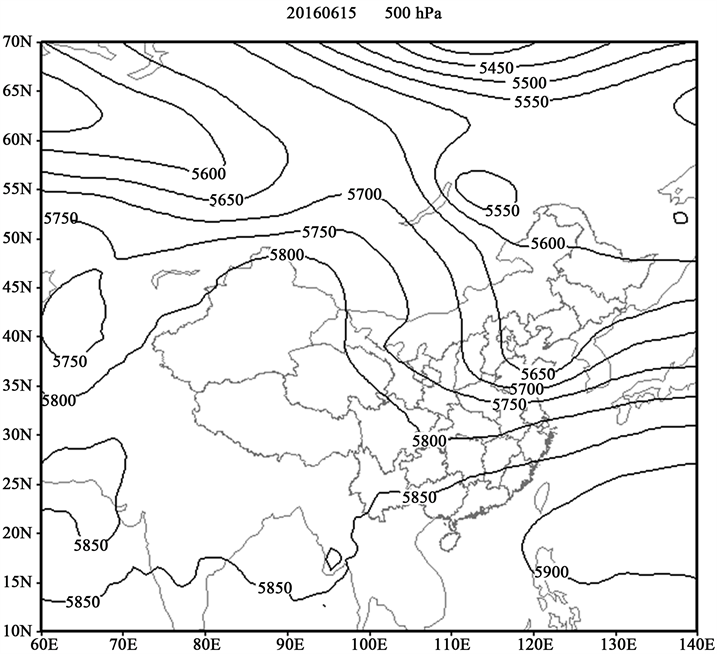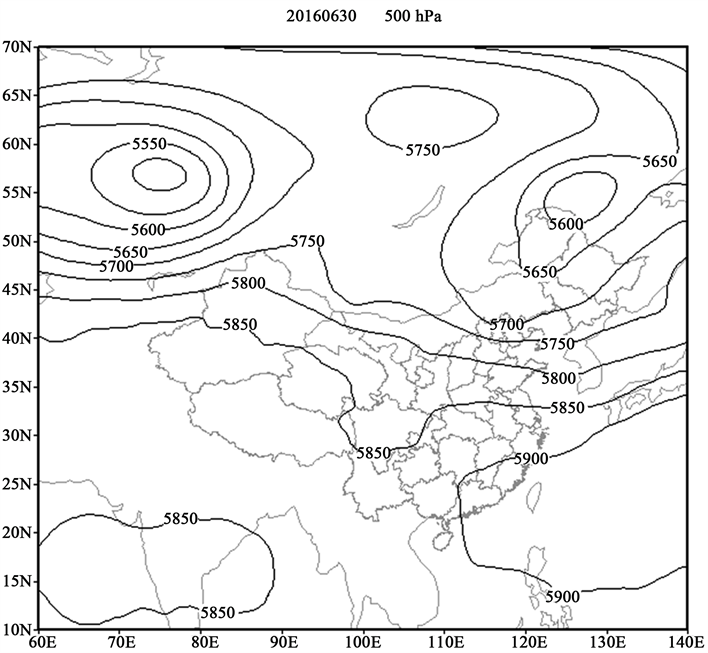Figure 1. The situation of Asia-Europe circulation in June

14日强降水带主要在长江以北地区，强降水中心位于江苏北部，至15日南移至长江以南，强中心位于湖南和江西北部，长江中下游部分地区降水量达到50 mm，达到大雨级别。至16日，雨带南移至两广地区，17，18日华南沿海地区强降水较为明显，至19日，降水带北移，长江中下游一带有较强降水产生，其中，湖北和安徽南部均有大于30 mm的降水产生，局地超过50 mm，已达到暴雨级别，降水强中心位于湖北湖南交界处；20日，我国南方的降水区域被分成两部分，其中一个大值区位于湖南一带，另一个大值区移动到江苏，江苏大部降水量超过25 mm，局地超过60 mm，为大雨至暴雨级别(图2)。

4. 长江中下游波能分布特征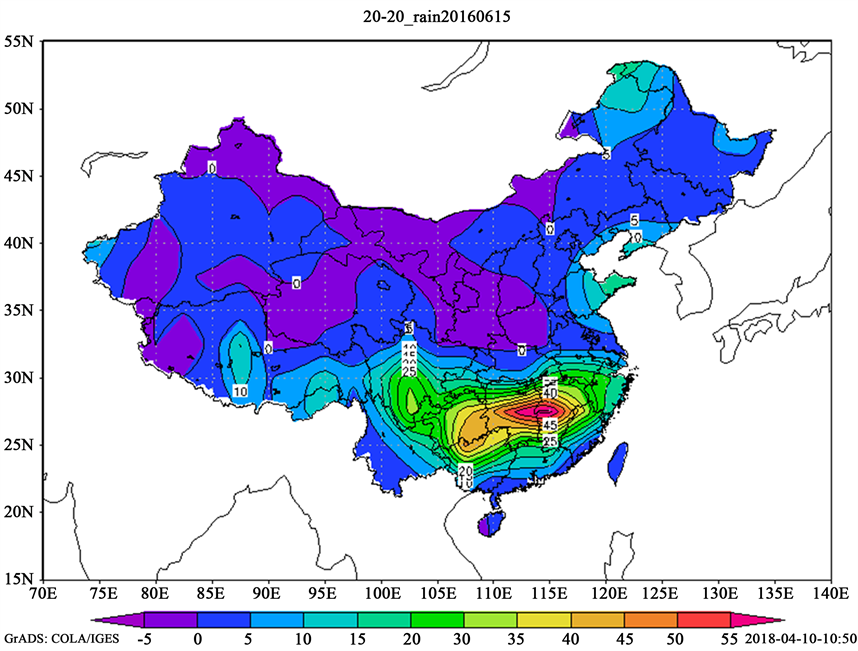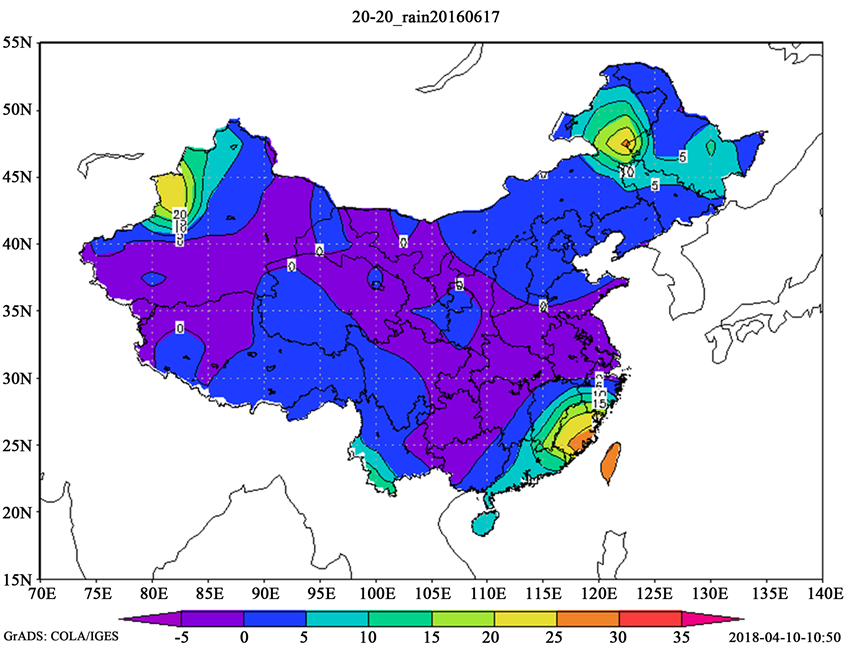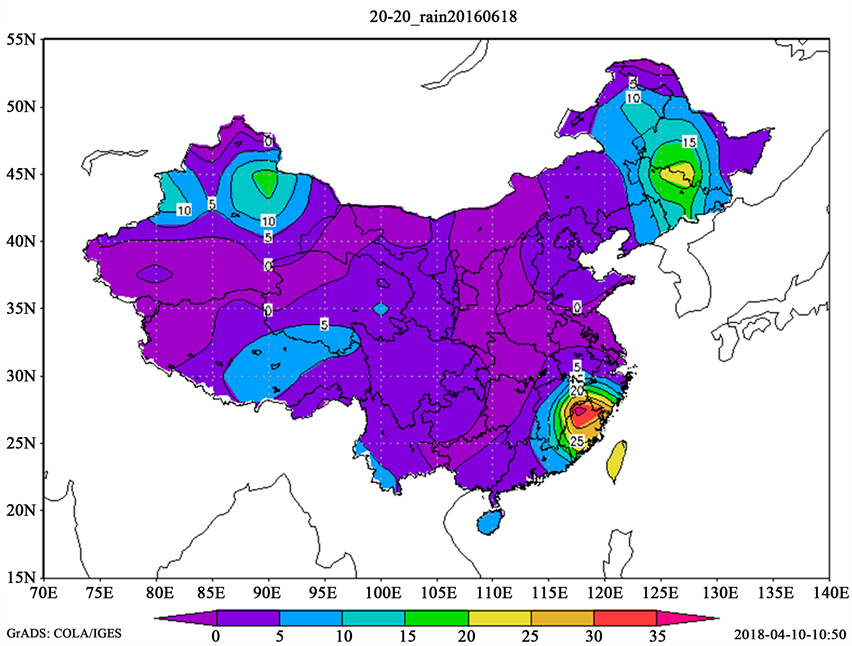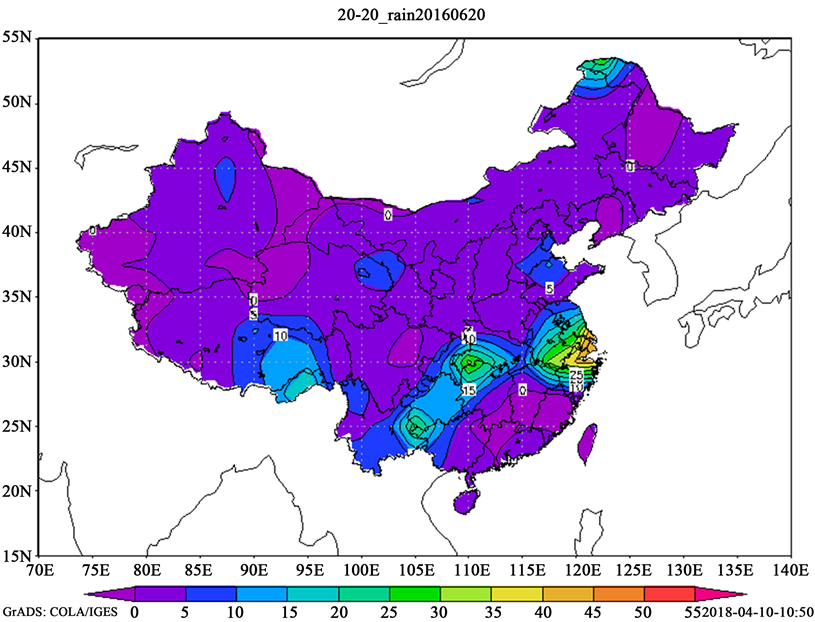Figure 2. Spatial distribution of precipitation during June 15~20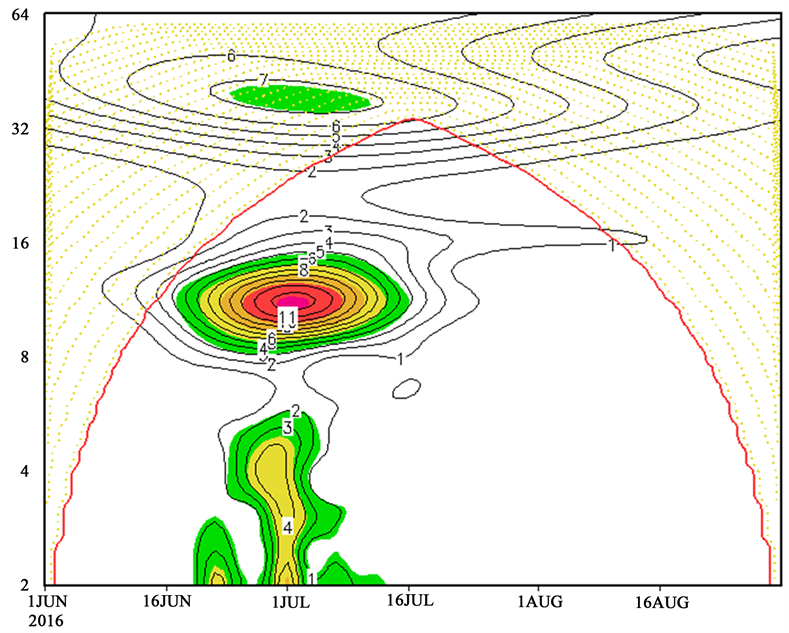Figure 3. Spatial average wavelet analysis of June-August precipitation in Jiangsu province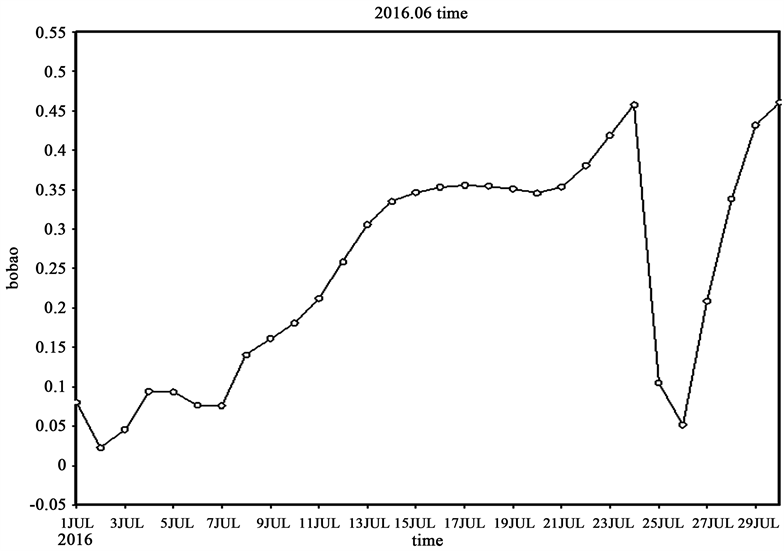Figure 4. Time series diagram of 500 hPa wave packet value in June

5. 扰动能量的积累和传播特征

5.1. 降水期间扰动能量演变特征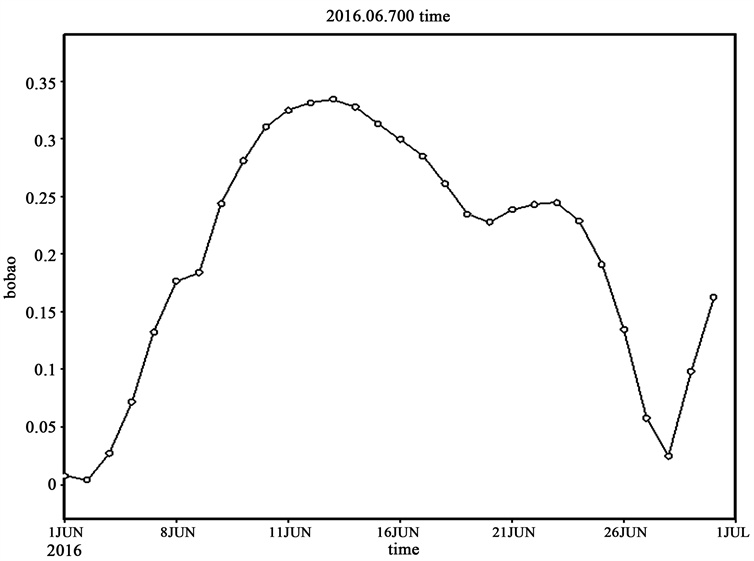Figure 5. Time series diagram of 700 hPa wave packet value in June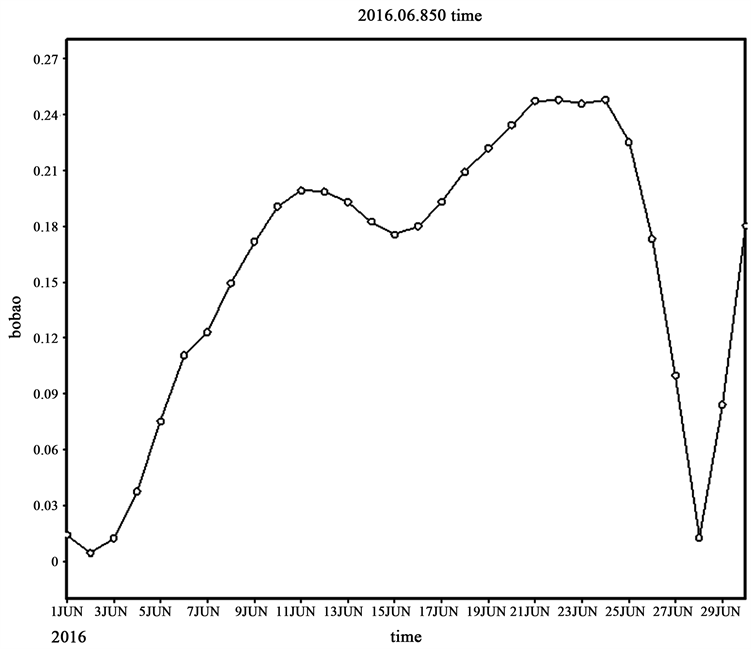Figure 6. Time series diagram of 850 hPa wave packet value in June

5.2. 扰动能量经向传播特征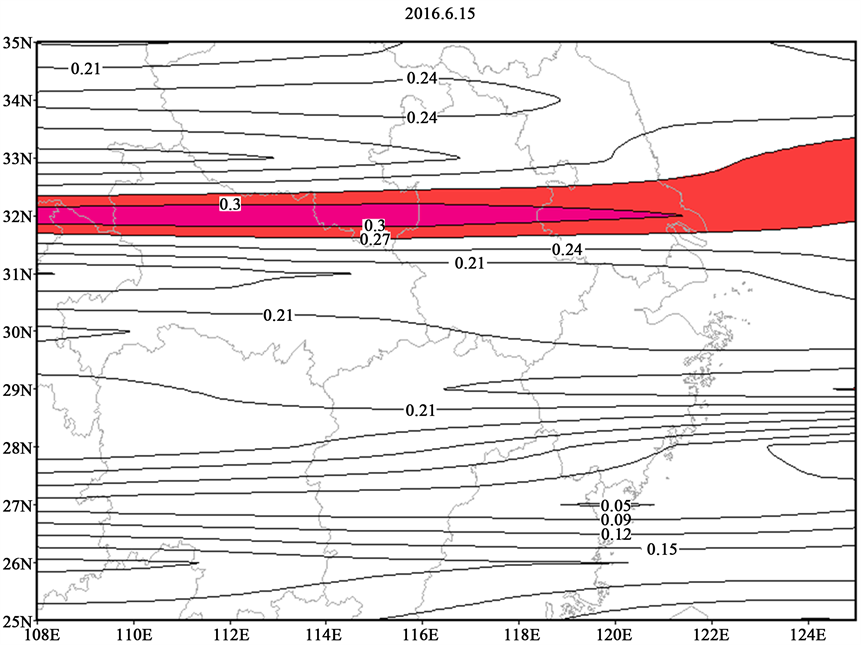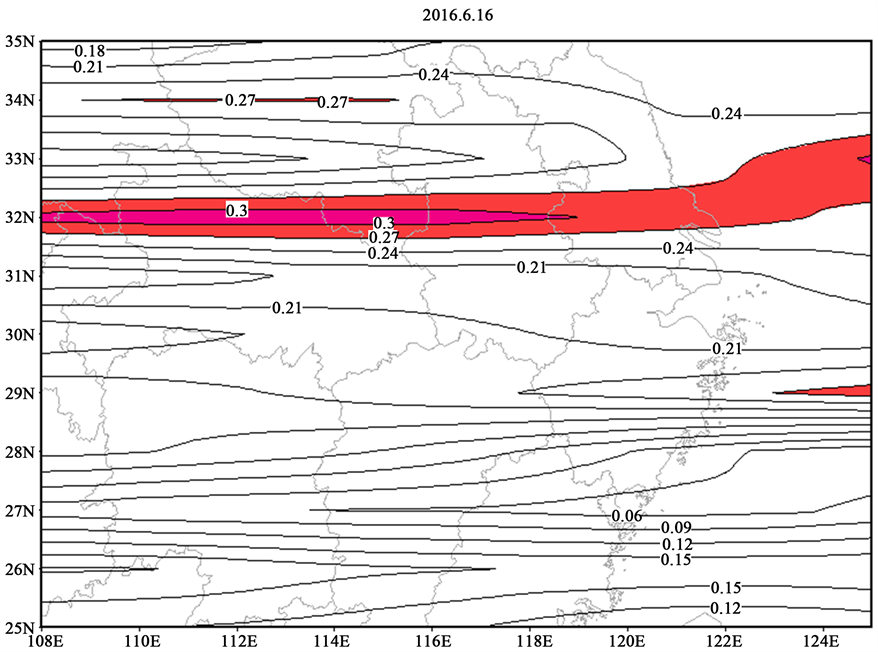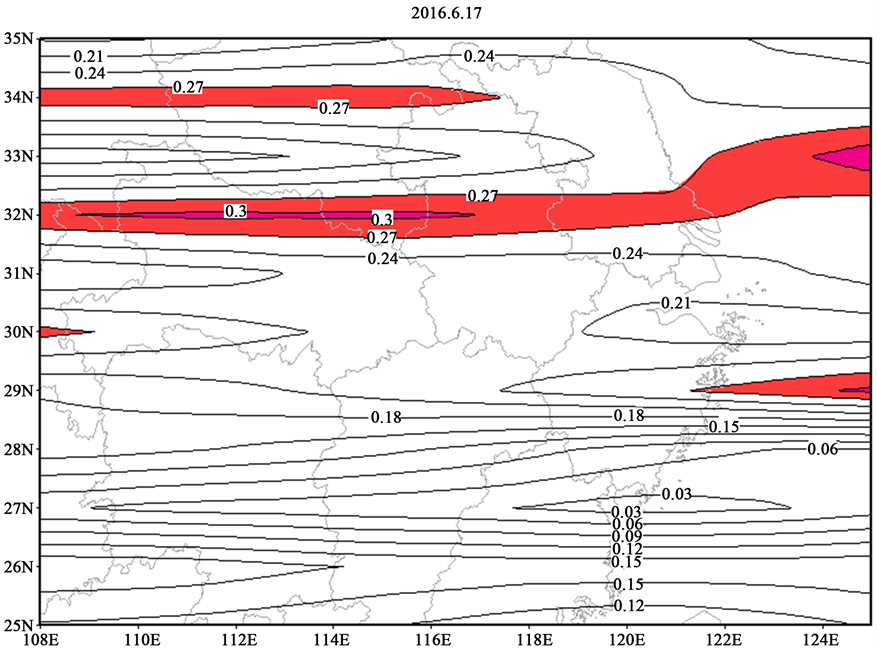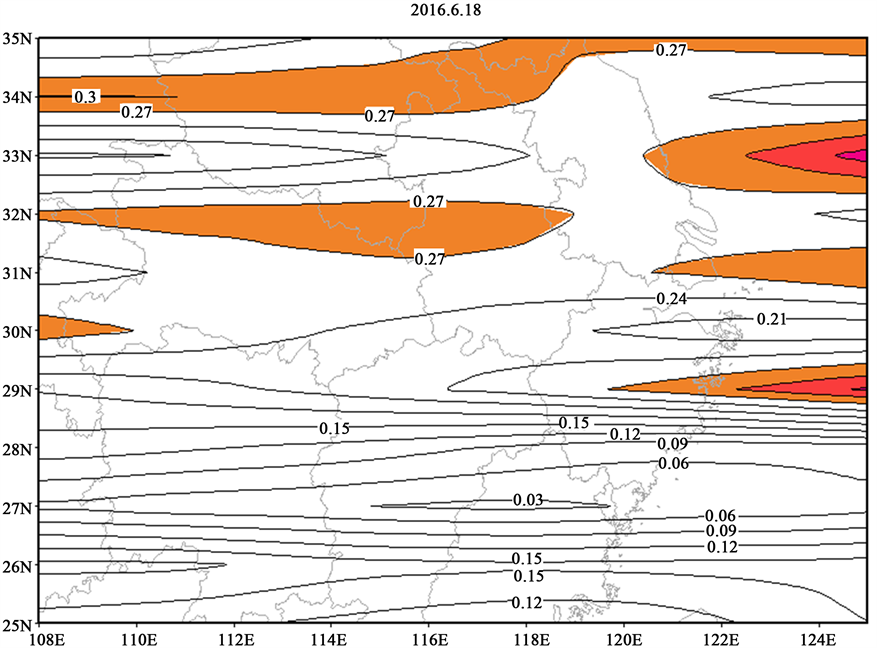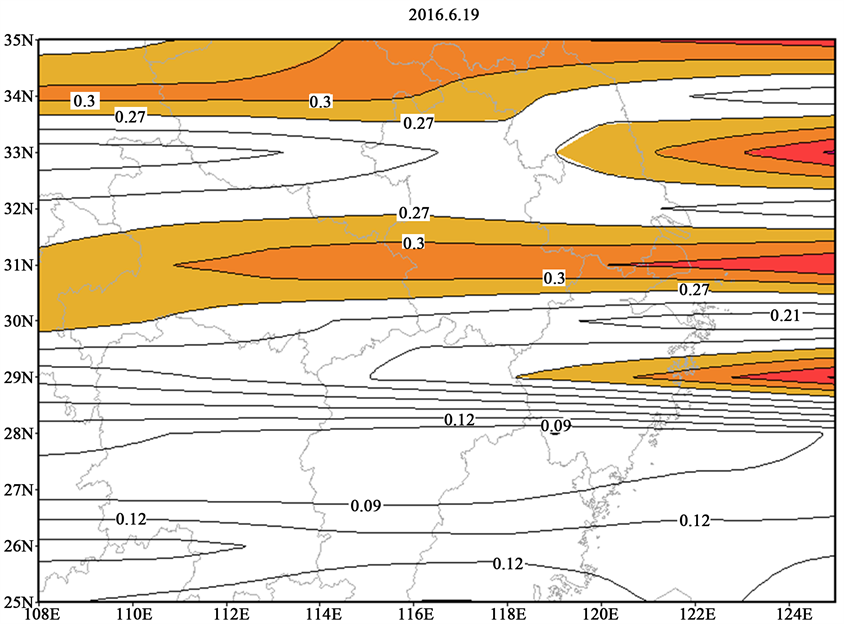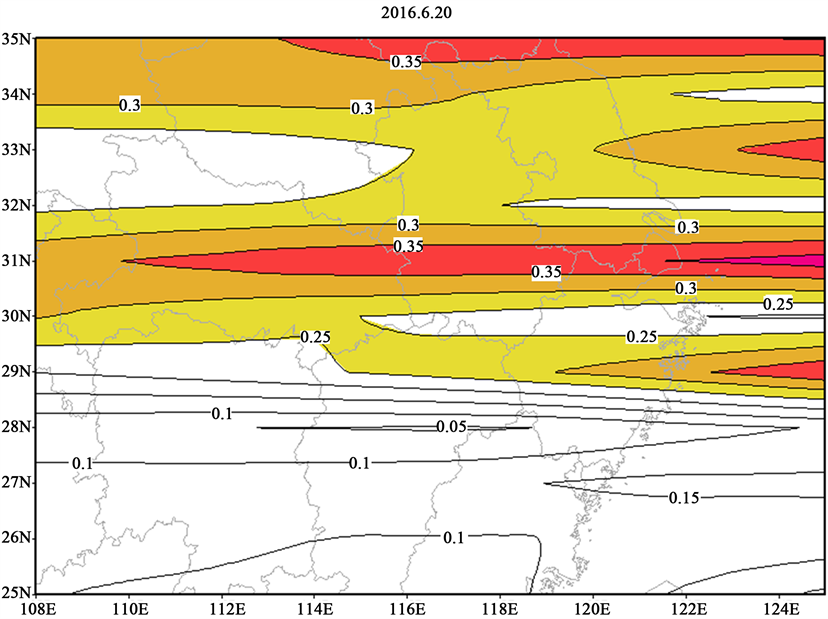Figure 7. Spatial distribution of wave envelope values from June 15 to June 20

5.3. 扰动能量纬向传播特征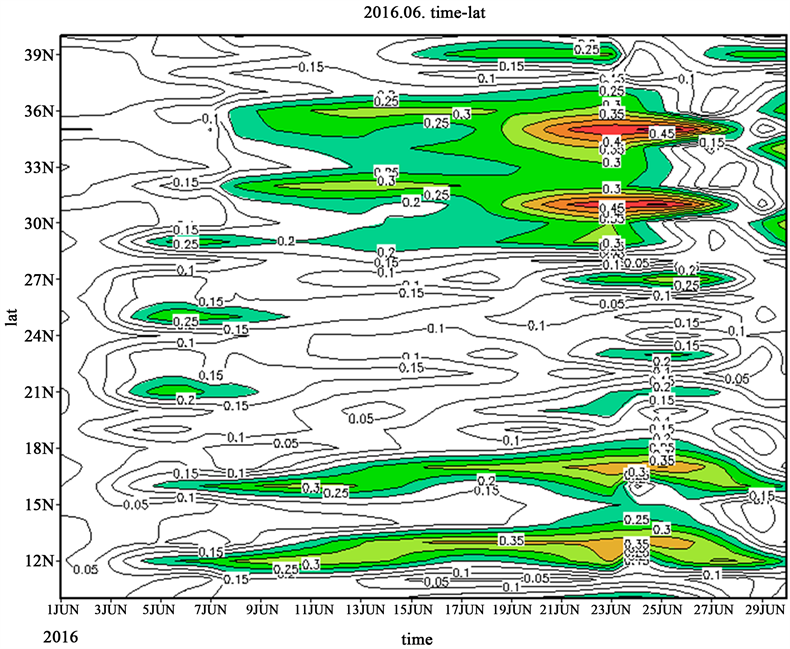Figure 8. June wave energy transversal profile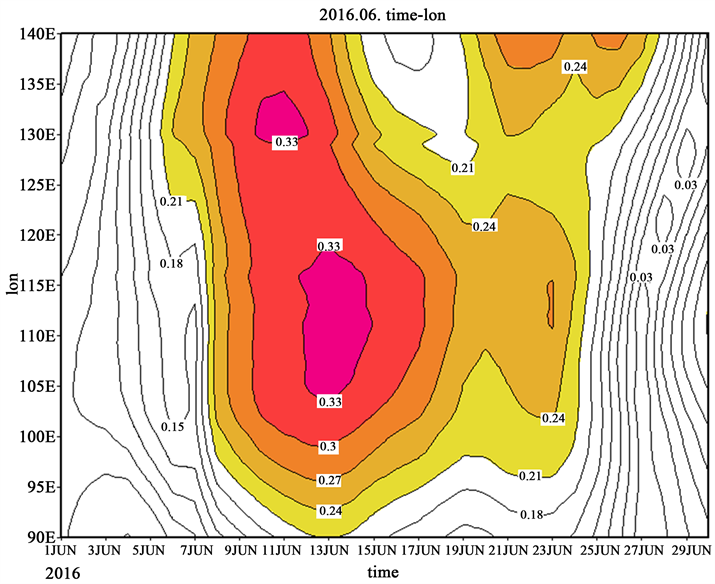Figure 9. June wave energy zonal profile

6. 结论

1) 6月发生在长江中下游地区的强降水天气过程基本特征为强度强，持续时间长。

2) 2016年初夏，欧亚大陆中高纬度主要呈两脊一槽或两槽一脊环流形势，冷空气在我国以北地区活动频繁，初夏长江中下游降水的基本机制即为副高携带暖湿气流和北方冷空气的共同作用。

3) 850 hPa受地形影响，对降水的影响更为滞后，500 hPa的周期显著性更高。

4) 在强降水过程发生期间，降水区域附近有强波表示的较强的扰动能量，且在降水过程期间维持在较为稳定的大值区，强波包能量在降水区域达到有利于降水条件时，即形成能量释放，为降水提供动力，且不断的波包传播与能量的释放也为降水维持提供了条件。

5) 通过分析15~30天周期的中频波代表的扰动能量的不断变化，发现中频波的传播以及扰动能量的不断变化对中长期天气变化有很好的指示作用。

NOTES

*通讯作者。

  毕宝贵, 张小玲, 代刊. 2016年超强厄尔尼诺背景下的强对流和极端降水天气特点[J]. 科学通报, 2017(9): 928-937.  倪允琪, 周秀骥, 张人禾, 等. 我国南方暴雨的试验与研究[J]. 应用气象学报, 2006, 17(6): 690-704.  吴玉明, 杨红卫. 南京市2016年暴雨洪水分析与思考[J]. 江苏水利, 2016(12): 69-72.  吴曼丽, 陆忠艳, 王瀛. 中期延伸天气预报方法研究[J]. 气象与环境学报, 2007, 23(2): 6-10.  张智勇. 欧洲中心和日本数值预报格点产品解释应用初探[J]. 吉林气象, 2005(1): 10-12.  Yeh, T.C. (1949) On Energy Dispersion in the Atmosphere. Journal of Meteorology, 6, 1-6. https://doi.org/10.1175/1520-0469(1949)006<0001:OEDITA>2.0.CO;2  曾庆存. 强迫基流上Rossby波包的结构和演变[J]. 中国科学, 1985, 15(4): 377-395.  Zeng, Q.C. (1983) The Evolution of Rossby Wave Packet in a Three Dimensional Baroclinic Atmosphere. Journal of the Atmospheric Sciences, 40, 73-84. https://doi.org/10.1175/1520-0469(1983)040<0073:TEOARW>2.0.CO;2  缪锦海, 肖天贵, 刘志远. 波包传播诊断的理论基础和计算方法[J]. 气象学报, 2002, 60(4): 461-467.  宋燕, 缪锦海, 琚建华. 波包传播特征与西太平洋副热带高压移动的关系[J]. 气象学报, 2006, 64(5): 577-582.  李湘, 肖天贵, 向洋. 1998年东亚夏季风波包传播特征研究[J]. 成都信息工程学院院报, 2007, 22(3): 365-368.  陈伟斌, 肖天贵, 谌芸, 等. 重庆“7.17”暴雨过程的波包分布及传播特征[J]. 暴雨灾害, 2009, 28(2): 126-130.  葛非, 肖天贵, 金荣花, 等. 2008年低温雨雪天气扰动能量的积累和传播[J]. 气象, 2008, 34(12): 11-20.  段鹤, 夏文梅, 苏晓力, 等. 短时强降水特征统计及临近预警气象[J]. 气象, 2014, 40(10): 1194-1206.  何冬燕, 邓学良, 赵勇. 初春青藏高原地表温度变化对长江中下游降水的影响[J]. 高原气象, 2010, 29(3): 579-586.  周宁芳, 康志明, 赖芬芬. 年夏季华北降水和环流形势的低频振荡特征分析[J]. 气象, 40(9): 1106-1113.  Murakami, T. (1979) Temperature Changes over Eurasia during the Late Summer of 1979. Advances in Atmospheric Sciences, 2, 200-214.  吕宏忠, 张先恭, 丁一汇. 赤道地区向西传播的40天周期低频波[J]. 气象学报, 1991, 49(1): 29-38.  曹艳察, 张涛. 2016年6月大气环流和天气分析[J]. 气象, 2016, 42(9): 1154-1160.  权婉晴, 何立富. 2016年7月大气环流和天气分析[J]. 气象, 2016, 42(10): 1283-1288.  向纯怡, 许映龙. 2016年8月大气环流和天气分析[J]. 气象, 2016, 42(11): 1418-1424.